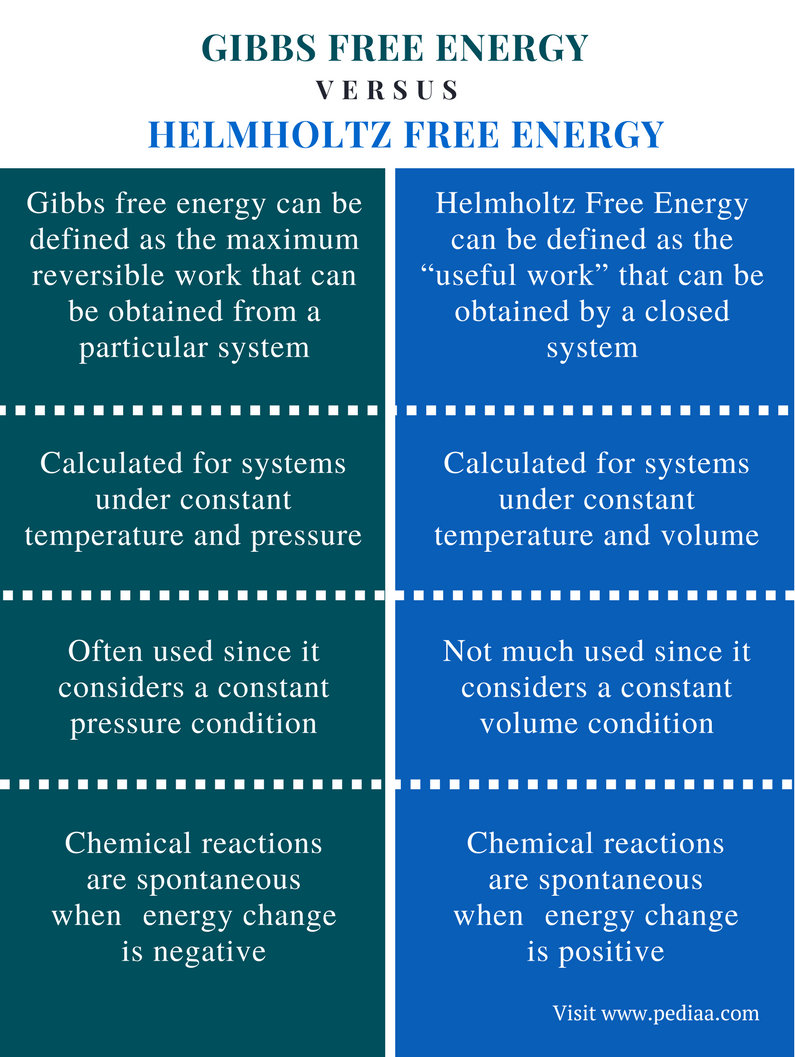# Difference Between Gibbs and Helmholtz Free Energy

## Main Difference – Gibbs vs Helmholtz Free Energy

There are four major thermodynamic potentials that are used in the thermodynamics of chemical reactions. They are internal energy, enthalpy, Helmholtz Free Energy and Gibbs free energy. Internal energy is the energy associated with the movement of molecules. Enthalpy is the total heat content of the system. Helmholtz Free Energy is the “useful work” that can be obtained from the system. Gibbs free energy is the maximum reversible work that can be obtained from a system. All these terms describe the behavior of a particular system. The main difference between Gibbs and Helmholtz free energy is that Gibbs free energy is defined under constant pressure while Helmholtz free energy is defined under constant volume.

### Key Areas Covered

1. What is Gibbs Free Energy
– Definition, Equation for Calculation, and Applications
2. What is Helmholtz Free Energy
– Definition, Equation for Calculation, and Applications
3. What is the Difference Between Gibbs and Helmholtz Free Energy
– Comparison of Key Differences

Key Terms: Enthalpy, Gibbs Free Energy, Helmholtz Free Energy, Internal Energy, Thermodynamic Potentials## What is Gibbs Free Energy

Gibbs free energy can be defined as the maximum reversible work that can be obtained from a particular system. In order to calculate this Gibbs free energy, the system should be at a constant temperature and constant pressure. The symbol G is given for Gibbs free energy. The Gibbs free energy can be used to predict whether a chemical reaction is spontaneous or non-spontaneous.

The Gibbs free energy is calculated from SI unit J (Joules). The Gibbs free energy gives the maximum amount of work done by a closed system instead of expanding the system. The actual energy that fits this definition can be obtained when the reversible process is considered. Gibbs free energy is always calculated as the change of energy. This is given as ΔG. This is equal to the difference between the initial energy and the final energy. The equation for the Gibbs free energy can be given as below.

### Equation

#### G        =      U   –   TS   +   PV

Where, G is the Gibbs free energy,

U is the internal energy of the system,

T is the absolute temperature of the system,

V is the final volume of the system,

P is the absolute pressure of the system,

S is the final entropy of the system.

But, the enthalpy of the system is equal to the internal energy of the system plus the product of pressure and volume. Then, the above equation can be modified as below.

or

#### ΔG   =   ΔH   –   TΔS

If the value of ΔG is a negative value, that means, the reaction is spontaneous. If the value of ΔG is a positive value, then the reaction is non-spontaneous.Figure 1: An Exothermic Reaction

A negative ΔG indicates a negative ΔH value. That means the energy is released to the surrounding. It is called an exothermic reaction. A positive ΔG indicates a positive ΔH value. It is an endothermic reaction.

## What is Helmholtz Free Energy

Helmholtz Free Energy can be defined as the “useful work” that can be obtained by a closed system. This term is defined for a constant temperature and a constant volume. The concept was developed by the German scientist Hermann von Helmholtz. This term can be given in the below equation.

### Equation

#### A   =   U   –    TS

Where, A is the Helmholtz free energy,

U is the internal energy,

T is the absolute temperature,

S is the final entropy of the system.

For spontaneous reactions, ΔA is negative. Therefore, when a chemical reaction in a system is considered, the change in the energy that is at constant temperature and volume should be a negative value in order for it to be a spontaneous reaction.

## Difference Between Gibbs and Helmholtz Free Energy

### Definition

Gibbs Free Energy: Gibbs free energy can be defined as the maximum reversible work that can be obtained from a particular system.

Helmholtz Free Energy: Helmholtz Free Energy can be defined as the “useful work” that can be obtained by a closed system.

### Constant Parameters

Gibbs Free Energy: The Gibbs free energy is calculated for systems under constant temperature and pressure.

Helmholtz Free Energy: The Helmholtz free energy is calculated for systems under constant temperature and volume.

### Application

Gibbs Free Energy: The Gibbs free energy is often used since it considers a constant pressure condition.

Helmholtz Free Energy: The Helmholtz free energy is not much used because it considers a constant volume condition.

### Chemical Reactions

Gibbs Free Energy: Chemical reactions are spontaneous when the Gibbs free energy change is negative.

Helmholtz Free Energy: Chemical reactions are spontaneous when the Helmholtz free energy change is negative.

### Conclusion

Gibbs free energy and Helmholtz free energy are two thermodynamic terms used in describing the behavior of a system thermodynamically. Both these terms include the internal energy of the system. The main difference between Gibbs and Helmholtz free energy is that Gibbs free energy is defined under constant pressure, while Helmholtz free energy is defined under constant volume.

##### References:

1. “Helmholtz Free Energy.” Helmholtz and Gibbs Free Energies, Available here. Accessed 25 Sept. 2017.
2. “Gibbs free energy.” Wikipedia, Wikimedia Foundation, 12 Sept. 2017, Available here. Accessed 25 Sept. 2017.
3. “Helmholtz free energy.” Wikipedia, Wikimedia Foundation, 12 Sept. 2017, Available here. Accessed 25 Sept. 2017.

##### Image Courtesy:

1. “ThermiteReaction” By User:Nikthestunned (Wikipedia) – Own work – also at Flickr (CC BY-SA 3.0) via Commons Wikimedia